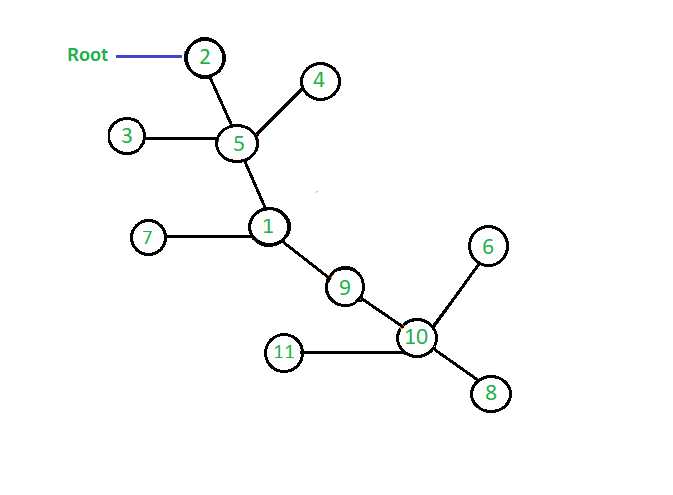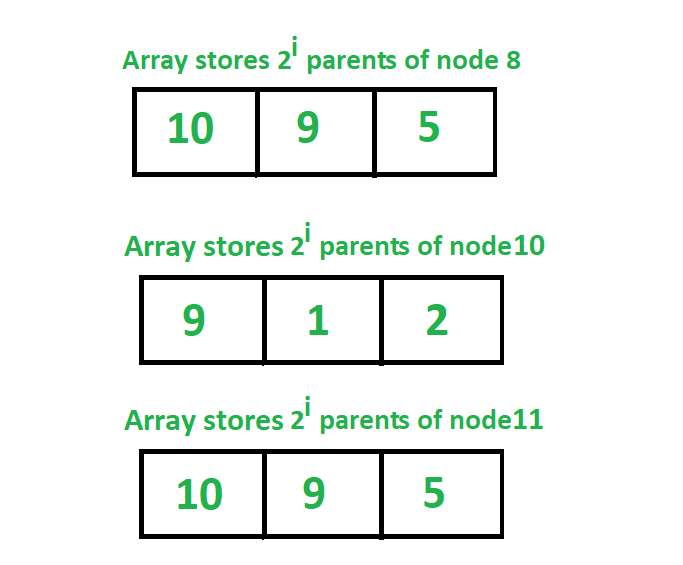Jump Pointer Algorithm

• Last Updated : 05 Nov, 2021

The Jump pointer algorithm is a design technique for parallel algorithms that operate on pointer structures, such as arrays or linked list. This algorithm is normally used to determine the root of the forest of a rooted tree.

In the jump pointer algorithm, we pre-process a tree so that one can able to answer the queries to find any parent of any node in the tree in time complexity of O(log n)

The jump pointer algorithm associates up to log2n pointers to each vertex of the tree. These pointers are called jump pointers because they jump up the tree towards the root node of the tree. For any node of the processing tree, the algorithm stores an array of length l jumpers where l = log2(depth(v)). The ith element of this array points to the 2ith parent of the node v. This data structure helps us to jump halfway up the tree from any given node. When the algorithm is asked to process a query to find any parent of any node in the tree, we repeatedly jump up the tree using these pointers. The number of jumps will be at most log n and therefore any problem to find the parent of any node in the tree can be answered in O(log n) time complexity.
In jump pointer, there is a pointer from node N to N’s j-th parent, for
j = 1, 2, 4, 8, …, and so on. So we store 2ith parent of each node.

The jump pointer algorithm basically works on the approach of dynamic programming where we use the pre-computed result to find the next result. By doing some simple calculations we can compute the mathematical formula that for any node k, 2jth parent of k is equal to 2j-1th parent of 2j-1th parent of k

The brief explanation of this formula is given below in the algorithm section.

The most common use of this algorithm is to solve the queries in which we need to find the ancestor of any node in O(log n) time complexity.

Graph to implement Jump Pointer Algorithm:Representation of jump array that stores 2^i th parent of all nodes:Examples:

Input: 0th parent of node 2
Output: 0th parent of node 2 is = 2

Input: 2th parent of node 4
Output: 2th parent of node 4 is = 2

Input: 3rd parent of node 8
Output: 3rd parent of node 8 is = 1

Algorithm :
Here is the algorithm to implement the jump pointer algorithm to find any parent of any node in the graph. We determine the jump matrix using dynamic programming. Here, we denote the root node as R and initially assume the parent of the root node as 0 which means there is no parent of this node. Now looking at the graph and the array is shown in the above diagram we can easily understand the above formula to determine the 2ith parent of each node. If we look at the node with value 8 we can see that its 20th parent is 10, now to find its 21th parent we see that it’s 21th parent is 20th parent of the node with value 10 and here 10 is 20th parent of 8 it means 21th parent of node 8 is 20th parent of 20th parent of node 8. Similarly, we can also see that 22th parent of node 8 is 5 which is 21th parent of 21th parent of node 8 i.e 21th parent of node with value 9
Thus in this way we can compute the array of jump pointers for all the nodes to store their 2ith parents.

Below is the pseudo code to calculate the jump pointer matrix that store 2ith parent of all the nodes in the tree.

jump[k][j] =   it points 2^jth parent of k
=  2^j-1th parent of (2^j-1th parent of k)
=  jump[jump[i][j-1][j-1]

Implementation: Below is the code to implement the above algorithm to find any parent of any node in O( logn ) time complexity.

Python3

Output:
0th parent of node 2 is = 2
2th parent of node 4 is = 2
3th parent of node 8 is = 1
Node is not present in graph

Time Complexity: O(logN) per query
Auxiliary Space: O(N * logN)

My Personal Notes arrow_drop_up